# 🔥「深入本质」一篇文章彻底理解递归

"递归"是所有语言都有的一种常用操作，但是你真的用好递归了吗？对递归没有任何疑问了吗？觉得递归总是找不到感觉不知道该怎么写吗？这篇文章正是为此而生的

• 什么是递归
• 如何选择停止条件
• 递归的本质、递归是如何工作的
• 面试题、递归应用实例

# 快速介绍

``````//最简单的理解，递归就是一个函数调用它自己
function blueFn(){
blueFn();
}

blueFn();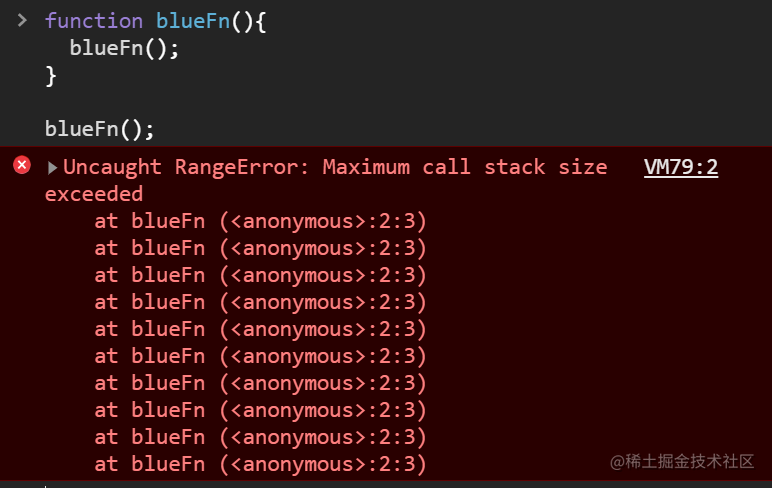## 递归的终止

``````function N(num){
//5! = 5 * 4!
return num*N(num-1);
}

N(5);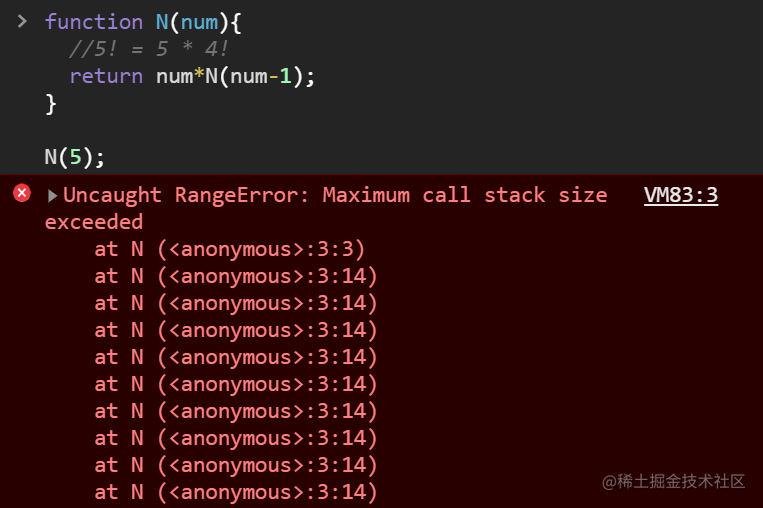``````function N(num){
if(num<=1)return 1;
else return num*N(num-1);
}

N(5);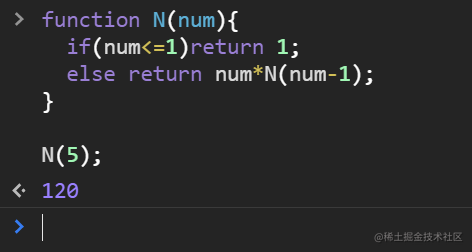• 函数调用其自身（直接的或间接的都算）
• 有明确的终止条件

# 什么是递归思想？

## 万物皆可分

• 库存清点：大型仓库如果一个人清点可能要好几个月才能完成
• 解决方案：找20个人各自负责一块，然后把清点结果汇总
• 数组求和：假设有有20G的数据需要求和，需要运行很久才能出结果
• 解决方案：找100台机器一起跑，每个负责200M，然后把各自的结果相加
• 搜索引擎：搜索引擎存储了数万亿页面，依靠单台服务器去找需要很久，但根据我们的经验，搜索结果0.x秒就能出来
• 解决方案：每台服务器负责一部分数据，然后将各部分搜索结果合并为最终的搜索结果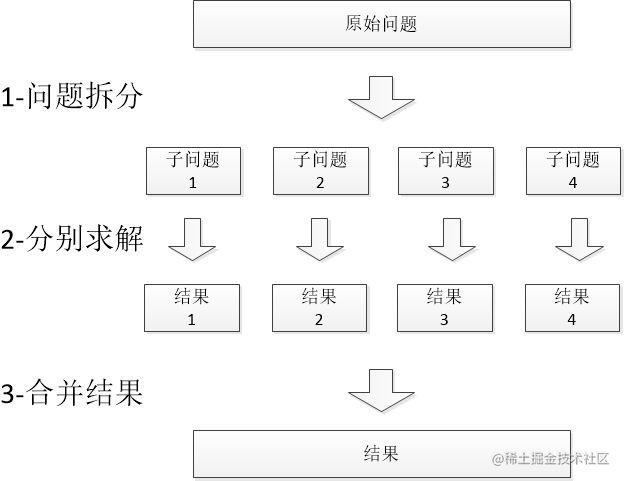## 实例理解递归

``````function findMax(arr){

}

• 首先，我们把数组分成左、右两半
• 找出左侧的最大值`maxL`，再找出右侧的最大值`maxR`
• 比较`maxL``maxR`，找到最终结果max
``````//注意：严格来说这个函数会有问题，需要判断arr.length才完整——暂略
function findMax(arr){
//终止条件：数组如果就1个值，它自己就是结果
if(arr.length==1)return arr;

//把数组分成左、右两半
const center=ceil(arr.length/2);  //取个整，因为下标不能是"2.5"这种
let arrL=arr.slice(0, center);
let arrR=arr.slice(center, arr.length);

//找出左侧的最大值`maxL`
let maxL=findMax(arrL);
//再找出右侧的最大值`maxR`
let maxR=findMax(arrR);

//比较`maxL`和`maxR`，找到最终结果max
if(maxL>maxR)
return maxL;
else
return maxR;
}

1. 把问题划分为更小的问题

``````let arrL=arr.slice(0, arr.length/2);
let arrR=arr.slice(arr.length/2, arr.length);
复制代码``````
2. 分别求解

``````let maxL=findMax(arrL);
let maxR=findMax(arrR);
复制代码``````
3. 把结果合并起来

``````if(maxL>maxR)
return maxL;
else
return maxR;
复制代码``````

## 递归是怎么执行的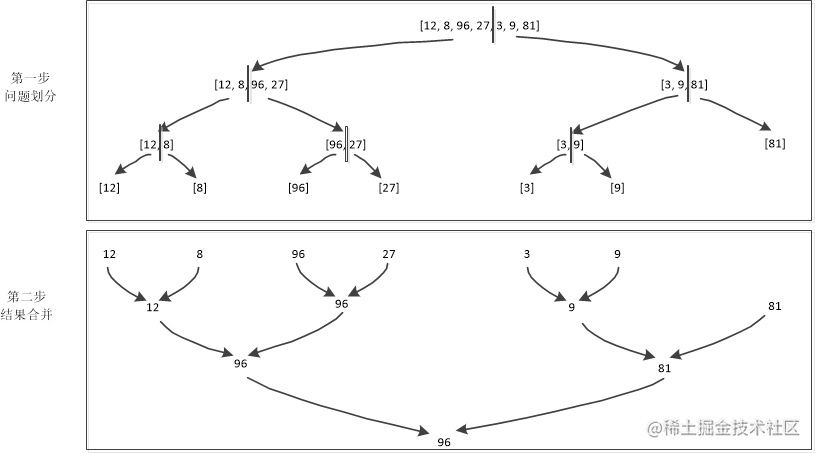• 第1次，我们会把它分成两部分：`[12, 8, 96, 27]``[3, 9, 81]`
• 并且，带来两个新的函数调用`findMax([12, 8, 96, 27])``findMax([3, 9, 81])`
• 然后，每个数组都会被进一步划分为两部分，直到不可再分为止

• 每次，`findMax`都会将两边的结果（例如：`12``8`）进行比较，并将其中较大的作为结果
• 重复这个过程，直到所有函数返回，既能得到最终结果

# 递归的本质、停止条件

• 为什么会栈溢出？什么是栈溢出？它咋就溢出了
• 我们应该如何选择终止条件？

## 递归的本质

### 函数调用栈

js和所有语言一样，内存中有一块专门存放函数调用的空间，称为“函数调用栈”，当我们调用函数或声明局部变量时，都是在操作它

``````function blueFn(num){
return sum(12, 5)+num;
}

function sum(a, b){
return a+b;
}

let res=blueFn(99);
console.log(res+8);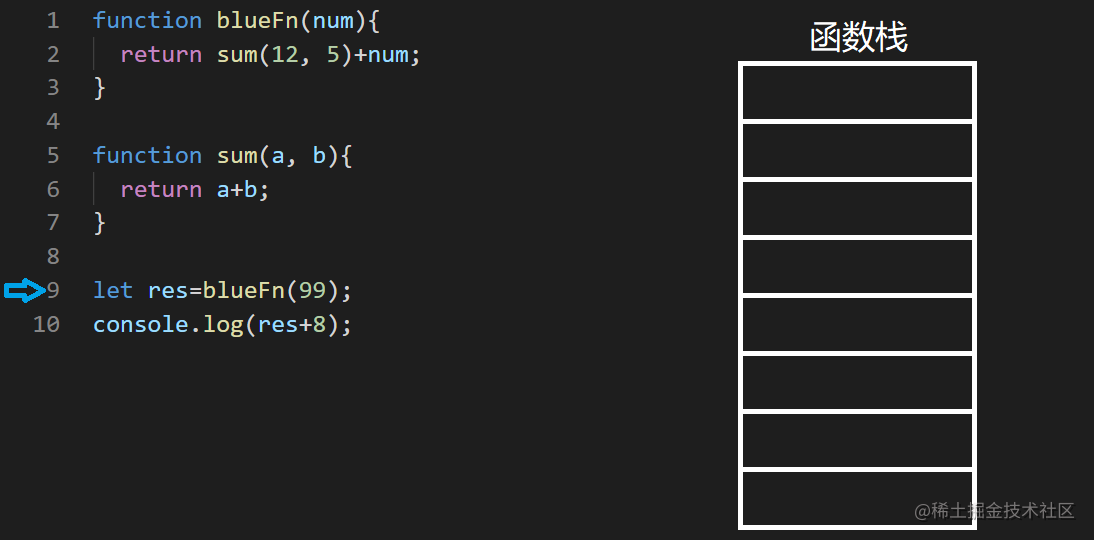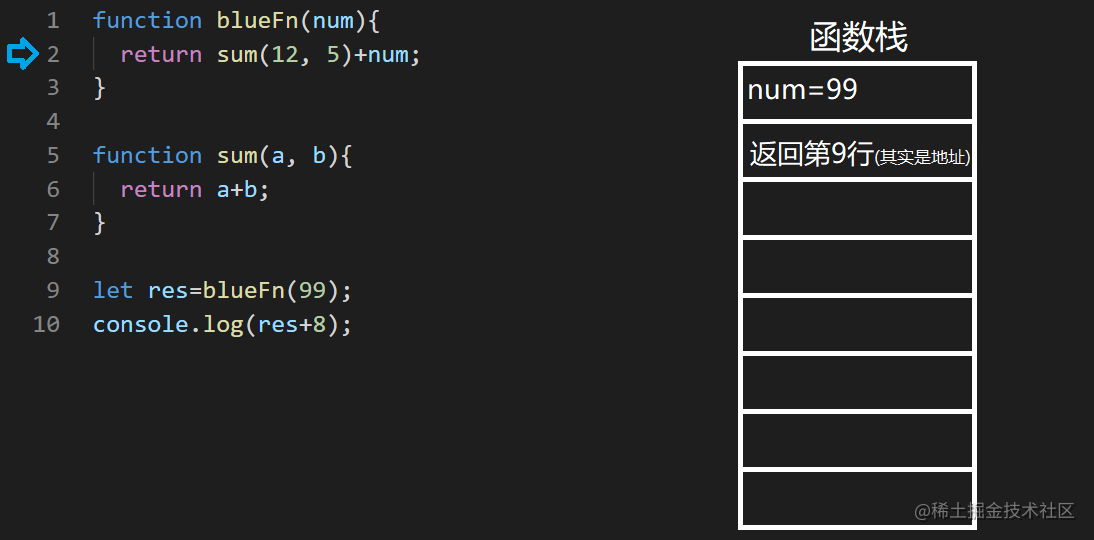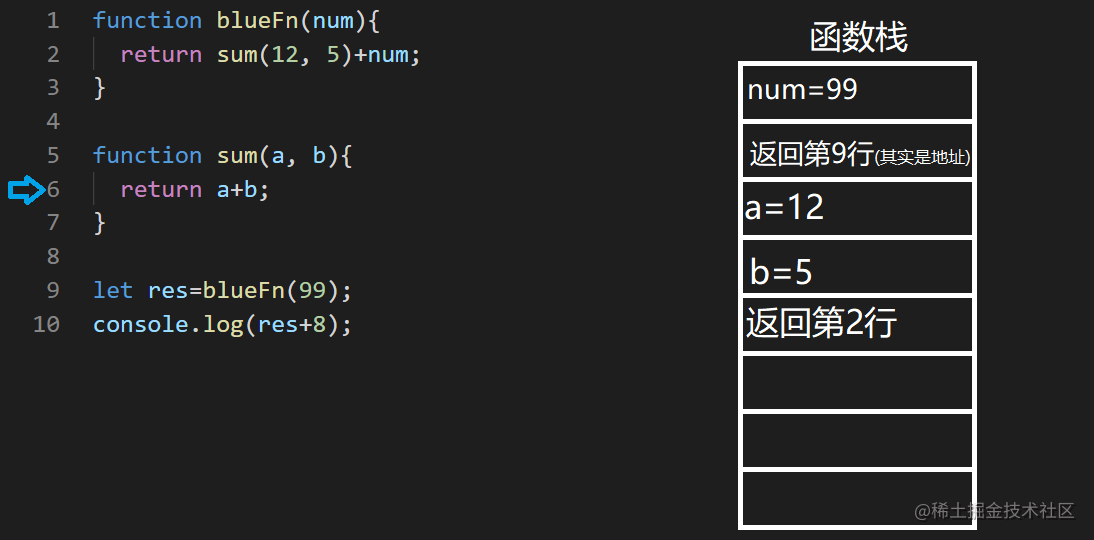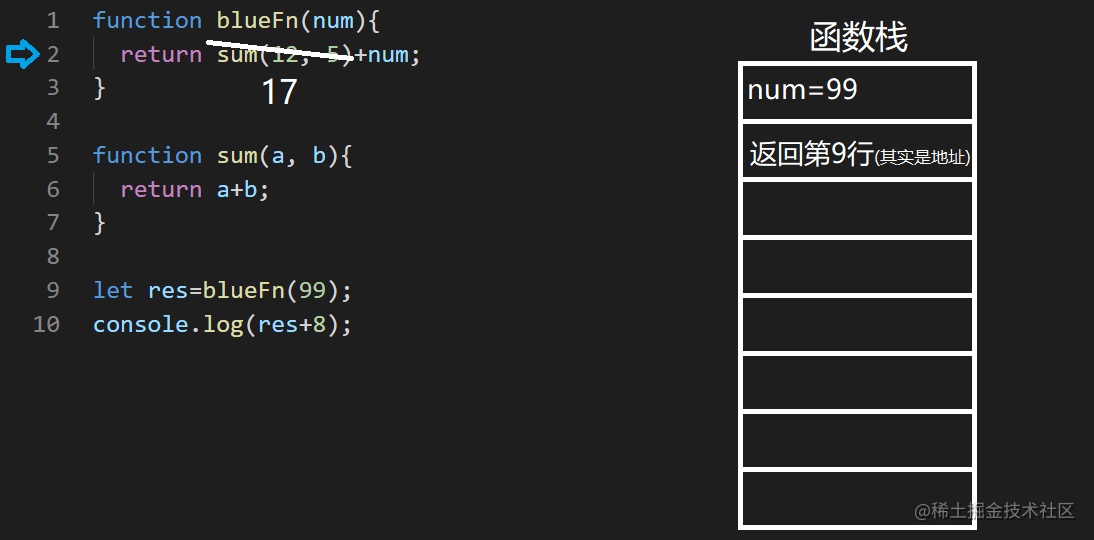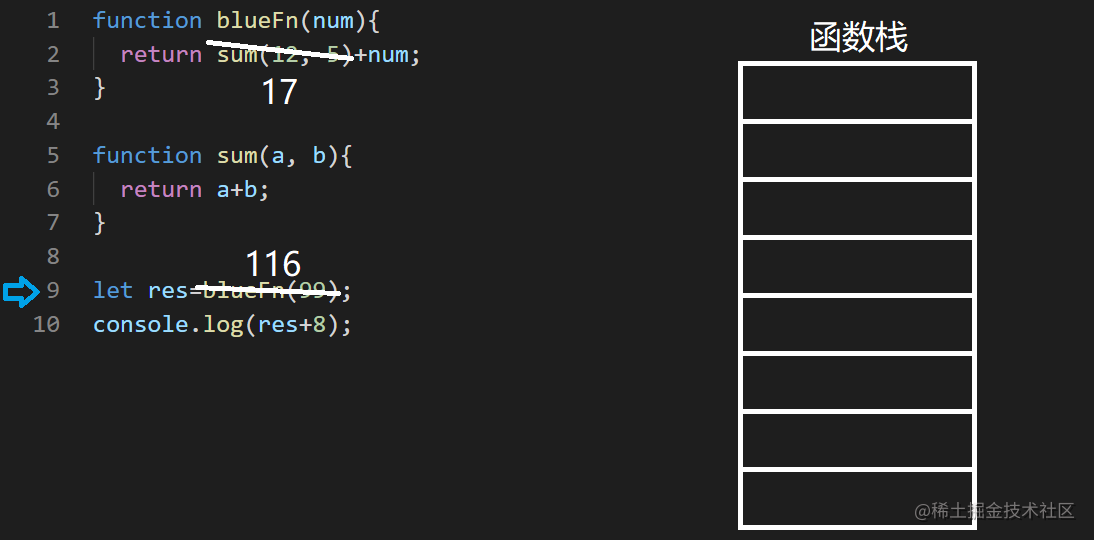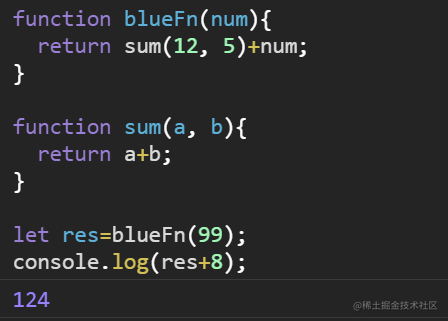• 函数的调用通过栈完成
• 函数的参数、局部变量、pc指针等，通过栈传递
• 函数调用层级越深，栈存储的东西越多

# 面试题、实例

## 题目1-数组的反转

``````let arr=[1,2,3];

function reverse(a){
//在这里具体写东西
}

reverse(arr);  //希望得到反转后的[3,2,1]

``````[1,2,3] -> [3,2,1]

//我们可以看做
1 和 [2,3] -> [3,2] 和 1

//也就是说
arr + arr[1...n] -> reverse(arr[1...n]) + arr

``````let arr=[1,2,3];

function reverse(a){
if(a.length<=1)return a;

const [first, ...rest]=a;
return [...reverse(rest), first];
}

reverse(arr);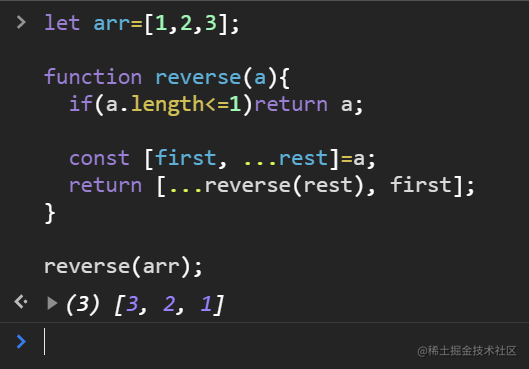## 题目2-不用循环遍历数组

``````function iterate(arr, callback){
//结束条件
if(arr.length==0)return;

//把数组分成第一个和其他
const [first, ...others]=arr;

//自己处理第一个
callback(first);
//剩下的递归
iterate(others);
}

# 总结• 狭义上，递归是指函数（直接或间接）调用它自身
• 广义上，递归是一种思想，我们可以把问题拆分为小块，分别求解，最后汇总得到整个问题的解
• 递归以及函数调用，本质上是在函数调用栈（call stack）中保存调用信息、参数，而调用栈有大小限制，超出会报错
• 递归的核心问题
• 如何拆分问题、分别求解、合并结果
• 如何选择合适的终止条件（重点）